Class 8 RD Sharma Solutions – Chapter 18 Practical Geometry – Exercise 18.2

• Last Updated : 05 May, 2021

Question 1: Construct a quadrilateral ABCD in which AB = 3.8 cm, BC = 3.0 cm, AD = 2.3 cm, AC = 4.5 cm and BD = 3.8 cm?

Solution:

We have, AB = 3.8 cm, BC = 3.0 cm, AD = 2.3 cm, AC = 4.5 cm and BD = 3.8 cm.

Step 1: Draw a line segment AC = 6cm.

Step 2: Taking A as the centre, draw an arc of radius = 3.8 cm. Mark that point as B.

Step 3: Taking C as the centre, draw an arc of radius = 3 cm intersecting point B.

Step 4: Taking B as the centre, draw an arc of radius = 3.8 cm. Mark that point as D.

Step 5: Taking A as the centre, draw an arc of radius = 2.3 cm intersecting point D.

Step 6: Now join AB, BD, AD, and DC to form a quadrilateral.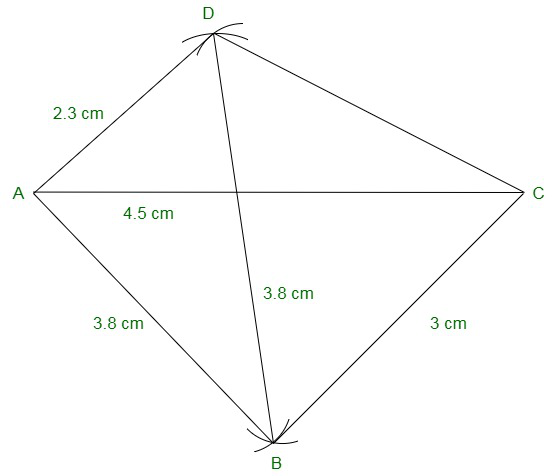Question 2: Construct a quadrilateral ABCD in which BC = 7.5 cm, AC = AD = 6 cm, CD = 5 cm and BD = 10 cm?

Solution:

We have, BC = 7.5 cm, AC = AD = 6 cm, CD = 5 cm and BD = 10 cm.

Step 1: Draw a line segment AC = 6cm.

Step 2: Taking A as the centre, draw an arc of radius = 6 cm. Mark that point as D.

Step 3: Taking C as the centre, draw an arc of radius = 5 cm intersecting point D.

Step 4: Taking D as the centre, draw an arc of radius = 10 cm. Mark that point as B.

Step 5: Taking C as the centre, draw an arc of radius = 7.5 cm intersecting point B.

Step 6: Now join AD, CD, DB, and AB to form a quadrilateral.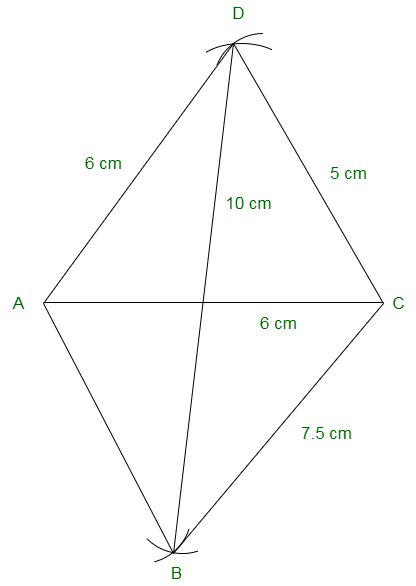Question 3: Construct a quadrilateral ABCD when AB = 3 cm, CD = 3 cm, DA = 7.5 cm, AC = 8 cm and BD = 4 cm?

Solution:

We have, AB = 3 cm, CD = 3 cm, DA = 7.5 cm, AC = 8 cm and BD = 4 cm.

Consider a triangle ABD,

Now, AB + BD = 3 + 4 = 7 cm

Note: Sum of lengths of two sides of a triangle is always greater than the third side.

∴ The construction is not possible.

Question 4: Construct a quadrilateral ABCD given AD = 3.5 cm, BC = 2.5 cm, CD = 4.1 cm, AC = 7.3 cm and BD = 3.2 cm?

Solution:

We have, AD = 3.5 cm, BC = 2.5 cm, CD = 4.1 cm, AC = 7.3 cm and BD = 3.2 cm.

Step 1: Draw a line segment CD = 4.1cm

Step 2: Taking C as the centre, draw an arc of radius = 7.3 cm. Mark that point as A.

Step 3: Taking D as the centre, draw an arc of radius = 3.5 cm intersecting point A.

Step 4: Taking D as the centre, draw an arc of radius = 3.2 cm. Mark that point as B.

Step 5: Taking C as the centre, draw an arc of radius = 2.5 cm intersecting point B.

Step 6: Now join CA, DA, DB, CB, and AB to form a quadrilateral.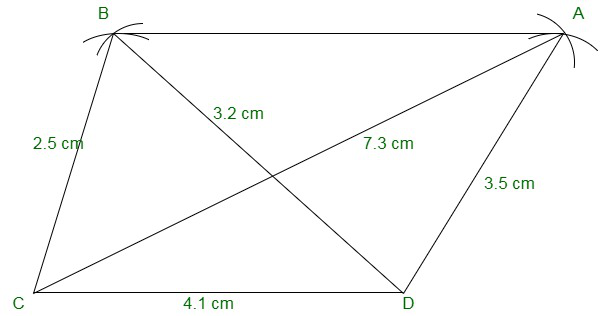Question 5: Construct a quadrilateral ABCD given AD = 5 cm, AB = 5.5 cm, BC = 2.5 cm, AC = 7.1 cm and BD = 8 cm?

Solution:

We have, AD = 5 cm, AB = 5.5 cm, BC = 2.5 cm, AC = 7.1 cm and BD = 8 cm.

Step 1: Draw a line segment AB = 5.5 cm

Step 2: Taking B as the centre, draw an arc of radius = 2.5 cm. Mark that point as C.

Step 3: Taking A as the centre, draw an arc of radius = 7.1 cm intersecting point C.

Step 4: Taking B as the centre, draw an arc of radius = 8 cm. Mark that point as D.

Step 5: Taking A as the centre, draw an arc of radius = 5 cm intersecting point D.

Step 6: Join the line segments BC, AC, BD, AD, and CD to form a quadrilateral.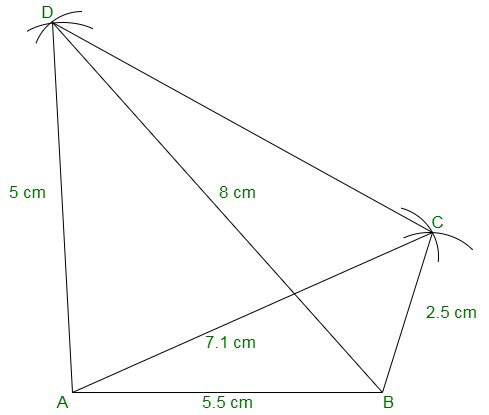Question 6: Construct a quadrilateral ABCD in which BC = 4 cm, CA = 5.6 cm, AD = 4.5 cm, CD = 5 cm and BD = 6.5 cm?

Solution:

We have, BC = 4 cm, CA = 5.6 cm, AD = 4.5 cm, CD = 5 cm and BD = 6.5 cm.

Step 1: Draw a line segment BC = 4cm

Step 2: Taking B as the centre, draw an arc of radius = 6.5 cm. Mark that point as D.

Step 3: Taking C as the centre, draw an arc of radius = 5 cm intersecting point D.

Step 4: Taking C as the centre, draw an arc of radius = 5.6 cm. Mark that point as A.

Step 5: Taking D as the centre, draw an arc of radius = 4.5 cm intersecting point A.

Step 6: Join the line segments BD, CD, CA, DA, and AB to form a quadrilateral.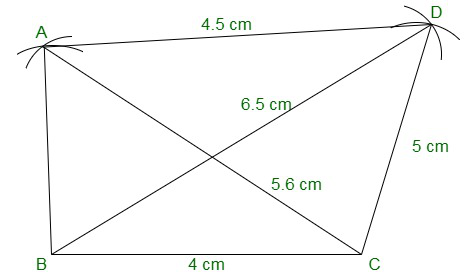My Personal Notes arrow_drop_up